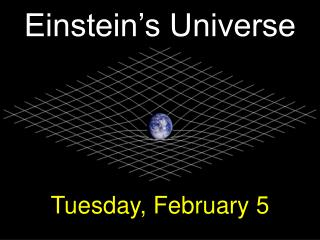Download PresentationEinstein’s Universe

# Einstein’s Universe

Download Presentation## Einstein’s Universe

- - - - - - - - - - - - - - - - - - - - - - - - - - - E N D - - - - - - - - - - - - - - - - - - - - - - - - - - -
##### Presentation Transcript

1. Einstein’s Universe Tuesday, February 5

2. Einstein – Newton smackdown! Two different ways of thinking about gravity and space.

3. The Way of Newton: Space is static (not expanding or contracting) and flat. (“Flat” means that all Euclid’s laws of geometry hold true.)

4. “Objects have a natural tendency to move on straight lines at constant speed.” However, we see planets moving on curved orbits at varying speed. How do you explain that, Mr. Newton?

5. “There is a force acting on the planets – the force called GRAVITY.” The gravitational force depends on a property that we may call the “gravitational mass”, mg.

6. Fg = gravitational force Mg = mass of one object mg = mass of other object r = distance between centers of objects G = “universal constant of gravitation” (G = 6.7 × 10-11 Newton meter2 / kg2)

7. Newton gave another law that gives the acceleration in response to any force (not just gravity)! The acceleration depends on a property that we may call the “inertial mass”, mi.

8. If a gravitational force is applied to an object with gravitational mass mg and inertial mass mi, its acceleration is

9. Truly astonishing and fundamental fact of physics: mg = mi for every known object! This equality is known as the “equivalence principle”.

10. The equivalence principle (which Newton leaves unexplained) led Einstein to devise his theory of General Relativity. Let’s do a “thought experiment”, of the kind beloved by Einstein.

11. Two ways of thinking about a bear: 1) Bear has constant velocity, box is accelerated upward. 2) Box has constant velocity, bear is accelerated downward by gravity.

12. Two ways of thinking about light: 1) Light has constant velocity, box is accelerated upward. 2) Box has constant velocity, light is accelerated downward by gravity.

13. Einstein’s insight: Gravity affects the paths of photons, even though they have no mass! Mass and energy are interchangeable: E = mc2

14. Einstein Newton Mass & energy are very different things. Mass & energy are interchangeable: E = mc2 Space & time are interchangeable: part of 4-dimensional space-time. Space & time are very different things.

15. Light takes the shortest distance between two points. In flat space, the shortest distance between two points is a straight line. In the presence of gravity, light follows a curved line. In the presence of gravity, space is not flat, but curved!

16. A third way of thinking about a bear: 3) No forces are acting on the bear; it’s merely following the shortest distance between two points in space-time.

17. The Way of Newton: Mass tells gravity how much force to exert; force tells mass how to move. The Way of Einstein: Mass-energy tells space-time how to curve; curved space-time tells mass-energy how to move.

18. Objects with lots of mass (& energy) curve space (& distort time) in their vicinity.

19. The Big Question: How is the universe curved on large scales (bigger than stars, bigger than galaxies, bigger than clusters of galaxies)? That depends on how mass & energy are distributed on large scales.

20. The cosmological principle: On large scales (bigger than stars, galaxies, clusters of galaxies) the universe is homogeneous and isotropic.

21. There are three ways in which space can have homogeneous, isotropic curvature on large scales. (Apologetic aside: describing the curvature of 3-dimensional space is difficult; I’ll show 2-d analogs.)

22. (1) This 2-d space is flat (or Euclidean):

23. (2) This 2-d space is positively curved:

24. (3) This 2-d space is negatively curved:

25. Measuring curvature is easy…in principle. Flat: angles of triangle add to 180° Positive: angles add to >180° Negative: angles add to <180°

26. Curvature is hard to detect on scales smaller than the radius of curvature. Flat = good approximation Flat = bad approximation

27. d a d = Sun-star distance a=Sun-Earth distance (1 A.U.) Parallax (flat space) August p p February p = a/d

28. Parallax (positivecurvature) August p p February p < a/d

29. Parallax (negativecurvature) August p p p > a/d As d→infinity, p→a/R February

30. Bright idea: The smallest parallax you measure puts a lower limit on the radius of curvature of negatively curved space. Hipparcos measured ‘p’ as small as 0.001 arcsec; radius of curvature is at least 1000 parsecs.

31. We need Bigger trianglesto measure the curvature accurately! d  L =L/d (flat) >L/d (positive) <L/d (negative)

32. In a negatively curved universe,

33. The most distant objects we can see are the hot & cold spots on the Cosmic Microwave Background.

34. Universe became transparent when it was 350,000 years old. The largest hot & cold spots are about 700,000 light years across. Hot & cold spots should be 1° across …if the universe is flat.

35. But we’ve measured the size of the hot & cold spots, and they are 1° across! Consistent with flat (Euclidean) space.

36. What Would Einstein Say? Density > critical density: Positive curvature Density < critical: Negative curvature Since our universe is close to flat, the density must be close to the critical density.

37. Thursday: Midterm Exam Bring your calculator and your favorite writing utensil.